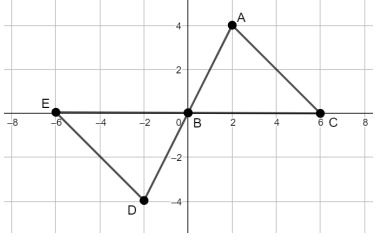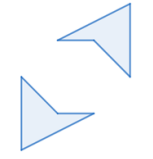# MA.912.GR.2.6Export Print
Apply rigid transformations to map one figure onto another to justify that the two figures are congruent.

### Clarifications

Clarification 1: Instruction includes showing that the corresponding sides and the corresponding angles are congruent.
General Information
Subject Area: Mathematics (B.E.S.T.)
Strand: Geometric Reasoning
Status: State Board Approved

## Benchmark Instructional Guide

### Terms from the K-12 Glossary

• Coordinate Plane
• Origin
• Reflection
• Rigid Transformation
• Rotation
• Translation

### Vertical Alignment

Previous Benchmarks

Next Benchmarks

### Purpose and Instructional Strategies

In grade 8, students identified a single transformation given the preimage and the image and learned that when the transformation is a reflection, a rotation or a translation, those transformations preserve congruence, that is, the preimage is mapped onto a copy of itself. In Geometry, students determine whether two figures are congruent and justify their answers using a sequence of rigid motions. This leads to the definition of congruence in terms of rigid transformations. (MTR.5.1)
• When identifying the transformations in the sequence, specify the vertical and horizontal translations, center and angle of the rotation, clockwise or counterclockwise, line of reflection, when needed.
• Instruction includes describing the rigid transformations using words and using coordinates.
• It is important to identify corresponding parts between the preimage and the image leading to the congruence statement and the congruence of the corresponding parts (angles and sides).
• Instruction includes using examples to compare transformations preserving angle measures and distance versus transformations just preserving angle measures. Include situations where the preimage and the image are not congruent to show how rigid motions will fail mapping one figure onto the other. (MTR.4.1)

### Common Misconceptions or Errors

• Students may have trouble seeing congruence when a reflection is needed because they limit their thinking to sliding a figure around without turning it over.

• Two triangles on the coordinate plane are shown below.• Part A. What transformation(s) could be applied to map triangle EBD onto triangle CBA
• Part B. Once the transformation is completed, how can you determine if the two triangles are congruent?

### Instructional Items

Instructional Item 1
• Describe the sequence of transformations that could be used to prove that the two quadrilaterals shown are congruent.*The strategies, tasks and items included in the B1G-M are examples and should not be considered comprehensive.

## Related Courses

This benchmark is part of these courses.
1200400: Foundational Skills in Mathematics 9-12 (Specifically in versions: 2014 - 2015, 2015 - 2022, 2022 and beyond (current))
1206310: Geometry (Specifically in versions: 2014 - 2015, 2015 - 2022, 2022 and beyond (current))
1206320: Geometry Honors (Specifically in versions: 2014 - 2015, 2015 - 2022, 2022 and beyond (current))
1206315: Geometry for Credit Recovery (Specifically in versions: 2014 - 2015, 2015 - 2022, 2022 and beyond (current))
7912065: Access Geometry (Specifically in versions: 2015 - 2022, 2022 and beyond (current))

## Related Access Points

Alternate version of this benchmark for students with significant cognitive disabilities.
MA.912.GR.2.AP.6: Use rigid transformations that includes translations or reflections to map one figure onto another to show that the two figures are congruent.

## Related Resources

Vetted resources educators can use to teach the concepts and skills in this benchmark.

## Formative Assessments

Justifying HL Congruence:

Students are asked to use rigid motion to explain why the HL pattern of congruence ensures right triangle congruence.

Type: Formative Assessment

Congruent Trapezoids:

Students will determine whether two given trapezoids are congruent.

Type: Formative Assessment

Transform This:

Students are asked to translate and rotate a triangle in the coordinate plane and explain why the pre-image and image are congruent.

Type: Formative Assessment

Proving the Alternate Interior Angles Theorem:

In a diagram involving two parallel lines and a transversal, students are asked to use rigid motion to prove that alternate interior angles are congruent.

Type: Formative Assessment

Showing Triangles Congruent Using Rigid Motion:

Students are asked to use the definition of congruence in terms of rigid motion to show that two triangles are congruent in the coordinate plane.

Type: Formative Assessment

Justifying SSS Congruence:

Students are asked to use rigid motion to explain why the SSS pattern of congruence ensures triangle congruence.

Type: Formative Assessment

Justifying SAS Congruence:

Students are asked to use rigid motion to explain why the SAS pattern of congruence ensures triangle congruence.

Type: Formative Assessment

Justifying ASA Congruence:

Students are asked to use rigid motion to explain why the ASA pattern of congruence ensures triangle congruence.

Type: Formative Assessment

## MFAS Formative Assessments

Congruent Trapezoids:

Students will determine whether two given trapezoids are congruent.

Justifying ASA Congruence:

Students are asked to use rigid motion to explain why the ASA pattern of congruence ensures triangle congruence.

Justifying HL Congruence:

Students are asked to use rigid motion to explain why the HL pattern of congruence ensures right triangle congruence.

Justifying SAS Congruence:

Students are asked to use rigid motion to explain why the SAS pattern of congruence ensures triangle congruence.

Justifying SSS Congruence:

Students are asked to use rigid motion to explain why the SSS pattern of congruence ensures triangle congruence.

Proving the Alternate Interior Angles Theorem:

In a diagram involving two parallel lines and a transversal, students are asked to use rigid motion to prove that alternate interior angles are congruent.

Showing Triangles Congruent Using Rigid Motion:

Students are asked to use the definition of congruence in terms of rigid motion to show that two triangles are congruent in the coordinate plane.

Transform This:

Students are asked to translate and rotate a triangle in the coordinate plane and explain why the pre-image and image are congruent.

## Student Resources

Vetted resources students can use to learn the concepts and skills in this benchmark.

## Parent Resources

Vetted resources caregivers can use to help students learn the concepts and skills in this benchmark.